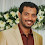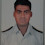# What is Indicated Power, Shaft Power And Break Power calculation formula

Indicated Power or Indicated Horse Power BHP Definition
>Power produced on the cylinder because of the expansion of gas is called Indicated Horse Power, negating any friction, heat loss or entropy within the system.
>It is known as the theoretical value of power of a reciprocating engine.
>It is calculated from the pressure developed inside the cylinder, measured by an instrument called Engine indicator.

Indicated Horsepower Formula

Indicated power (Pi) = Pm * A * L * N

Pm -Mean indicated pressure

A - Area of Piston
L - Length of stroke
N -No.of power stroke/sec

In this formula we know the values of A,L and N, Pm (mean indicated pressure) can be calculated by the use of  engine indicator.

Brake Horsepower (Bp) or Shaft Horsepower(Sp)
> Power available at the propeller shaft is known as Shaft horsepower , as this measured by means of a break is known as break power.
>Only a part of indicated power is available at the shaft because of the various frictional losses such as piston ring,cross head, top end bearing etc

How Brake Horsepower is measured?

>> It is measured by applying a resistance torque as a brake on the shaft the heat  generated by the friction is carried away by circulating water.

Brake Horsepower Formula
Bp=2πNFR
N-revolution per second of the engine
F-Resisting force of break
R-Radius at which the force is applied

How  Shaft Horsepower(Sp) is measured?
>>Torsion meter is the instrument used to measure the torque of the engine shaft.

Shaft Horsepower(Sp) Formula Sp=2πNT
T- torque which is measured by torsion meter
N-revolution per second of the engine

>> Discussion is an exchange of knowledge It Make the Mariner Perfect.... Please Discuss below...

1.There is one error that occurs several times, and it is due to a language misunderstanding. The presentation above talks about BREAK horsepower, but the proper term is BRAKE horsepower.

The word BREAK refers to dividing something into two or more parts. Thus, we break a stick into two parts. The word BRAKE refers to retarding or stopping a motion. A brake is used to load an engine in order to measure the power output. Both words are pronounced exactly the same in spoken English, but their meanings are quite different.

1.2.Is brake power and shaft power the same?

1.No brake power is always more than shaft horse power.As there is some more losses occuring in the transmission line.

2.Mr. Nishant, please read on. I spoke to this very issue in the messages that follow below. With your line of thinking, one would have to ask about losses incurred between the engine and the brake (even if they were exceedingly small) and this sort of nit-picking can go on without end.

3.In most contexts, brake Hp and shaft Hp are understood to be the same quantity.

1.In ships with midship engines, shaft could be 50 meter long, supported by nearly 10 intermediate bearings. Power received near the stern tube (Delivered Power) would be less than power available near the flywheel (Brake Power). .....BTW "Break" and "Brake" are pronounced diffrently. Brake is pronounced with a longer Bray....

4.Let me expand a bit on the previous answer.

Consider first the concept of shaft power, either in Hp or in W. To calculate shaft power (SP), you need to know the torque (T) and the shaft speed (w), the latter in radian/sec. To get the shaft speed, w ---
and shaft power is
SP = w * T
or
[=] (rad/s)*(m-N) = m-N/s = J/s = W

To get shaft horse power, it is only necessary to convert ft-lb/s to hp.

Brake power (whether in ft-lb/s or W) is shaft power measured at the flywheel.

Thus if we measure power on an auxiliary drive geared off the flywheel, that shaft power measurement will be reduced by the efficiency of the gear drive.

5.The formula you gave above is for work done on the piston head and not indicated horsepower. The actual for for indicated horsepower is i.h.p = (PmLAN)/4560.86.
Thanks.

1.can you please explain from where you get the value 4560.86?

6.Would Mr. Bob Olik explain the difference he sees between power at the piston head and indicated power, please? I think that they are the same.

Also, where does the divisor 4560l.86 come from? A derivation of his formula would be helpful.

1.nicely explained dr. D

7.PkPlea anyone can tell about how we get 4560.86 valu

8.From the textbook Elements of Thermodynamics and Heat Transfer, 2nd ed., by Obert and Young, p. 93, we read
ihp = P*L*A*N/33000
where
ihp = indicated horsepower
P = indicated mean effective pressure (imep), psi
L = stroke length, inches
A = piston area, inches^2
N = number of cycles per minute
When we examine the units of P*L*A*N, it is clear that
P*L*A*N [=] (lb/in^2)*(in)*(in^2)*(1/min)
[=] lb-in/min
Now 1 Hp = 550 lb-ft/sec, so it is evident that the distance and time factors in P*L*A*N are not correct and some unit corrections are required. The 4560.86 divisor cited by Mr. Olik is evidently for some unspecified units system.

9.thanks forthe very useful discussion regarding how to measure the bhp of main engine

10.If torsion meter not fitted in vessel or torsion meter not working then pls advise how to calculate shaft power. Is it possible to find SP using FO consumption and Running hours.

11.With no torque indication, I see no way to calculate shaft power. Fuel oil consumption is irrelevant as is to total engine hours.